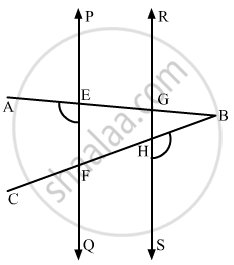# In the Given Figure, Pq || Rs, ∠Aef = 95°, ∠Bhs = 110° and ∠Abc = X°. Then the Value of X is - Mathematics

MCQ

In the given figure, PQ || RS, ∠AEF = 95°, ∠BHS = 110° and ∠ABC = x°. Then the value of x is•  15°

• 25°

• 70°

• 35°

#### Solution

In the given figure,

PQ || RSAlso,∠AEFand ∠1 are the corresponding angles.

Then, according to the Corresponding Angles Axiom, which states:

If a transversal intersects two parallel lines, then each pair of corresponding angles are equal.

Therefore,

∠1 = ∠AEF

It is given that

∠AEF = 95°

Therefore,

∠1 = 95°

Clearly, ∠1 and ∠2form a linear pair, therefore, their sum must be supplementary.

Therefore,

∠1 + ∠2 = 180°

On substituting ∠1 = 95°in equation above, we get:

95° +  ∠2 =180°

∠2 = 180° - 95°

∠2 = 85°

In ΔBHG:

We know that, in a triangle exterior angle is equal to the sum of the interior opposite angles. Therefore,

∠2+x = ∠BHS

Substituting  ∠BHS =110°

and ∠2 = 85°, we get :

85° + x = 110°

x = 110° - 85°

x = 25°

Is there an error in this question or solution?
Chapter 10: Lines and Angles - Exercise 10.6 [Page 53]

#### APPEARS IN

RD Sharma Mathematics for Class 9
Chapter 10 Lines and Angles
Exercise 10.6 | Q 13 | Page 53

Share